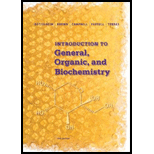# 5-47 The three main components of dry air and the percentage of each are N 2 (78.08%), O 2 (20.95%), and Ar (0.93%). (a) Calculate the mass of one mole of air. (b) Given the mass of one mole of air, calculate the density of air in g/L at STP.### Introduction to General, Organic a...

11th Edition
Frederick A. Bettelheim + 4 others
Publisher: Cengage Learning
ISBN: 9781285869759### Introduction to General, Organic a...

11th Edition
Frederick A. Bettelheim + 4 others
Publisher: Cengage Learning
ISBN: 9781285869759

#### Solutions

Chapter
Section
Chapter 5, Problem 5.47P
Textbook Problem

## Expert Solution

### Want to see the full answer?

Check out a sample textbook solution.See solution

### Want to see this answer and more?

Experts are waiting 24/7 to provide step-by-step solutions in as fast as 30 minutes!*

See Solution

*Response times vary by subject and question complexity. Median response time is 34 minutes and may be longer for new subjects.